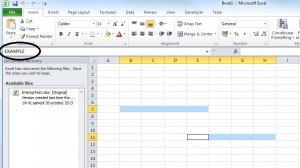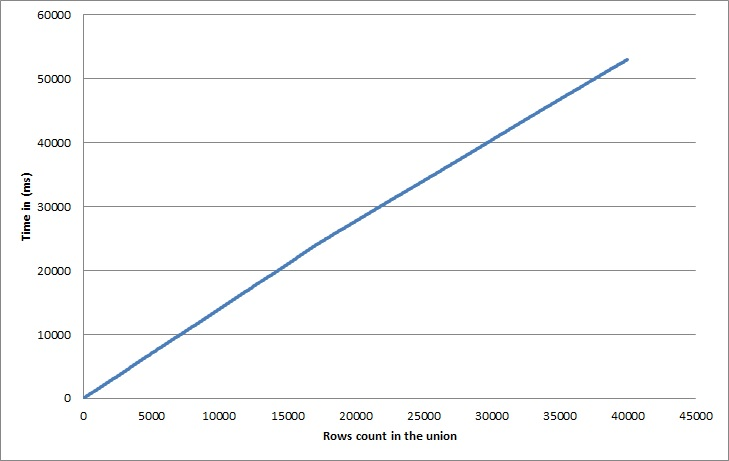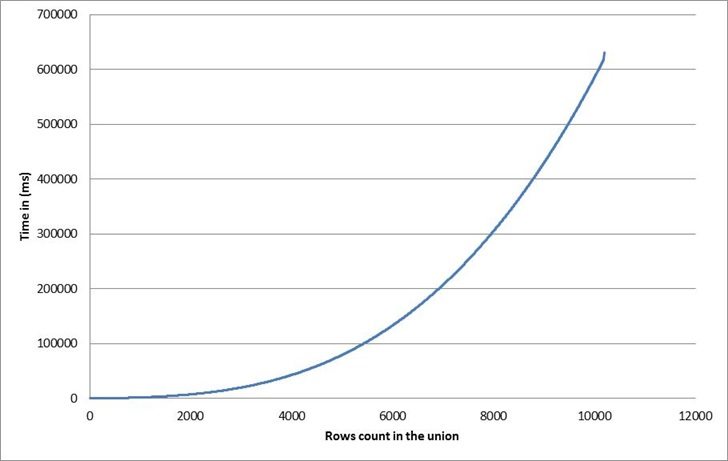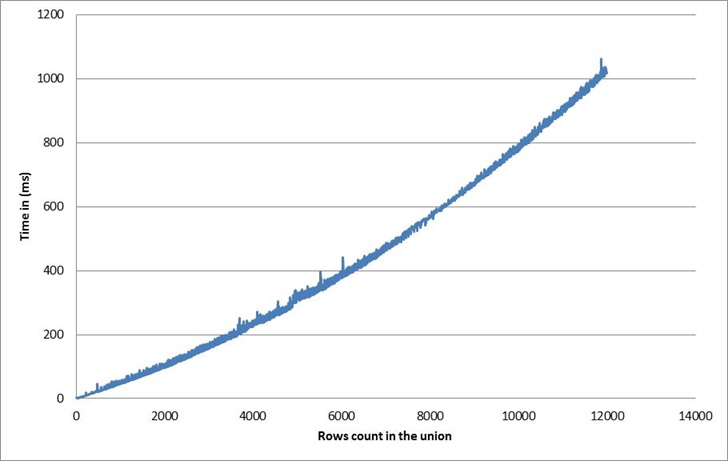My current task at work is the development of an excel addin. This addin is developed in C# .NET and is based on the Excel Interop assemblies, we also use Excel DNA for the packaging and the User Defined functions. While developing a new feature I stumbled upon a technical oddity of Excel Interop which I will describe to you in this post.

Let me start this post by reminding you that a range in Excel is not necessarily a block of contiguous cells. Indeed, try it yourself by starting excel right now. Then you can select a range, keep the Ctrl button of your keyboard press on and select an other block of contiguous cell. Then, you have several cells selected that you can name (as shown in the screenshot). Finally, you have created a named range with non-contiguous cells.A range of non-contiguous cells in Excel

Having said that, let us assume that for our addin we need to reference programmatically the range of all lines of the form, with usual excel notations, Ax:Cx where x describes a set of row indices.

Then we need to use the method Application.Union of Micorsoft.Office.Interop.Excel and finally produce few lines of code that  looks like that.

In the chart above we have monitored the time execution of the BuildUnionAll method for different values of the parameter count.Performance curve for the BuildUnion method

Remark: in the case of BuildUnionAll there is no need to use a loop and the Union method, we could have just ask for the range “A1:Ccount”. Note also that for small unions you may also use a syntax with semicolon to separate contiguous cells blocks e.g. worsheet.Range[“A1:C3;A8:C12”]. However, this does not work for large ranges made of multiple non-adjacent cells blocks.

So far so good, we see an almost linear curve, which is natural regarding to what we were expecting.

Now, change a little bit our expectation to something quite different and more realistic where we would truly need the Application.Union method. Then let us say that we would like to have the union Ax:Cx mentioned above but for x odd index only. We want the IEnumerable</em> to have the same size than before, so let us use the method in the code below.

Similarly, we monitor the performance curve.Quadratic performance

Argghhh!!! we get an awful quadratic cost which makes our approach completely broken for large unions. This quadratic cost was unexpected. Actually I did not found documentation on this (the msdn doc on Excel.Interop is really minimalist). However, it seems that the rule is that the Union method has a linear cost depending on the number of Areas in the input ranges. The Areas member of a Range is the collection containing the blocks of contiguous cells of the range.

This experimental rule, leads us to review our algorithm in a different way. If the cost of an Union is important (linear) when there are many areas in a range. Then we will try to minimize this situation: we will let our algorithm perform the union preferably on ranges having fewer areas. Once again, the basic techniques from school bring a good solution and we will design a very simple recursive algorithm based on the divide and conquer approach, inspired for the merge sort algorithm.

To the end, we recover an almost linear curve. The asymptotic complexity here (if the rows to pick are not adjacent to each other) equals the merge sort one which is O(n.ln(n)).Back to normal with divide and conquer approach